• Probabilistic quantities, represent and reason? Called Bayesian Networks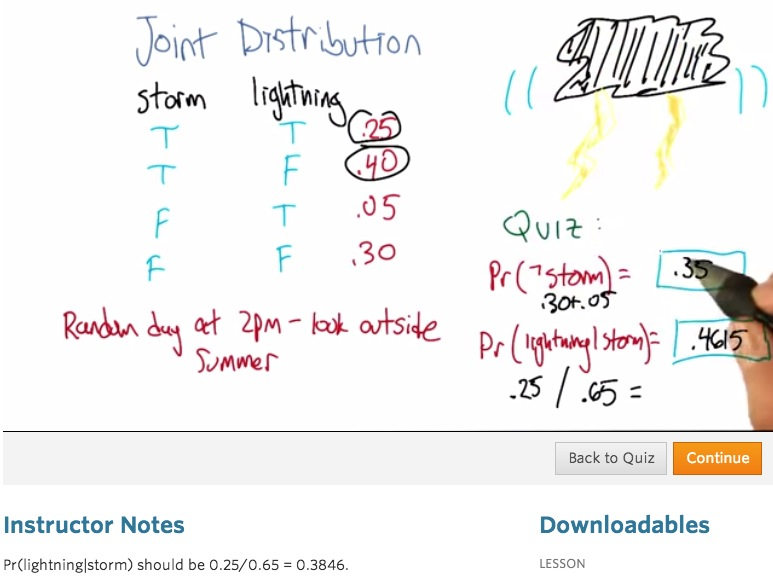• Since we this 2 event in the given data is independable, that's not a thing in Bayes, but for this possible data, we can answer some of the bayes' questions.
• When we look at the event storm not coming, we could directly look at the table which gives us accumulate probability of event occurs only False.
• Then as dependable, from given storm, we can infer the lighning happen in condition of probability that storm has occurs.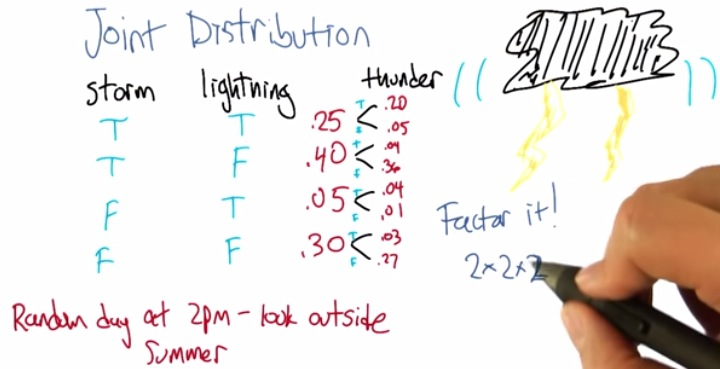• In for example, the world of binary classification only factor of two, which is efficient.
• But when it's comes to multiple classfification, the problem could be exponentially harder.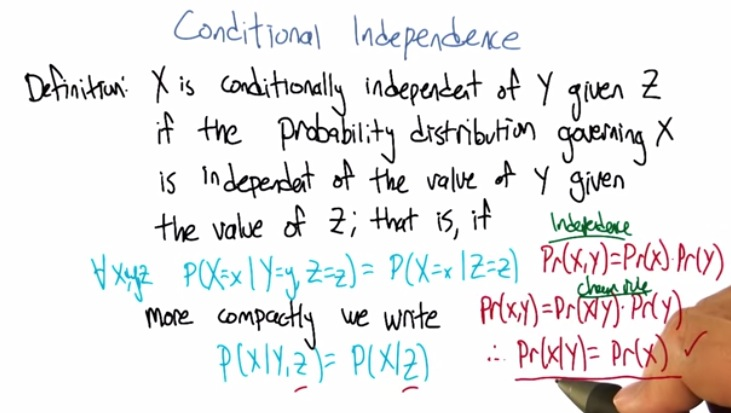• This means for all x,y,z, if value of x depend on value of y, but y is independent of value of z then x is conditionally independent of probability of y given z, we can safely ignore the y event and go directly to z, which infer the P(X|Z). As long as the probability of X|Y, is independent of.
• Independence, we can just multiply both probability.
• Based on chain rule, P(X|Y) = P(X) because both X and Y is independence.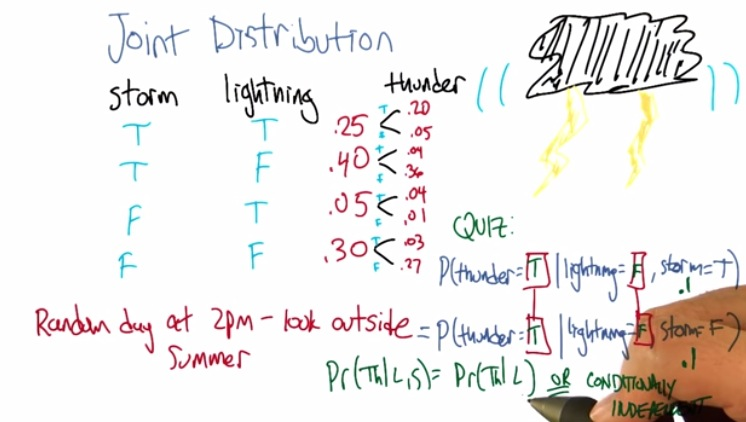• Given storm t/f , the probability of P(T|L)  must be the still the same.
• It's logical and simple explanation that, no matter what Storm happens, because it's independence, we simply can ignore the storm. Conditionally independent.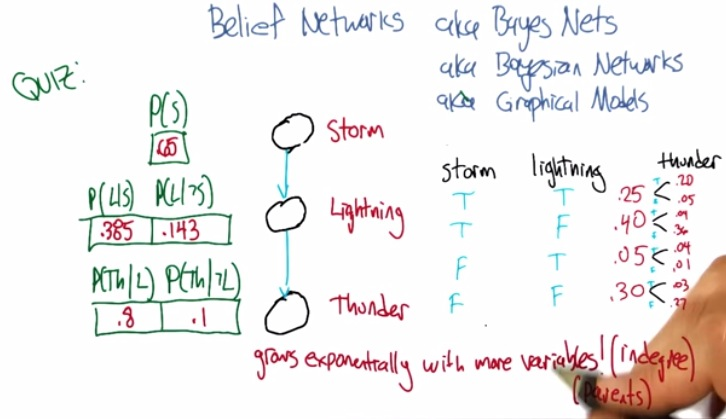• The first case is the probability of storm which simply accumulate where storm occurs.
• The second is probability which storm occurs, means that we count the probability of each L when S occurs.
• The third one is independence of S, which means, we get to choose between the two(storm or not storm) and count depend on lightning.
• This is the case when 2 dependence, 1 independence. But in complex, like in neural network, we're taking all possible combination(maybe 3 dependence) as long as the arrow goes to the thunder.
• This makes we have to count all the possible combination that goes into thunder.
• But in this case, we just take 2 dependance, 1 independence. KEEP IN MIND that the graph may show lightning depend on storm, and thunder depend on lightning, but it's not the case that thunder depend on storm.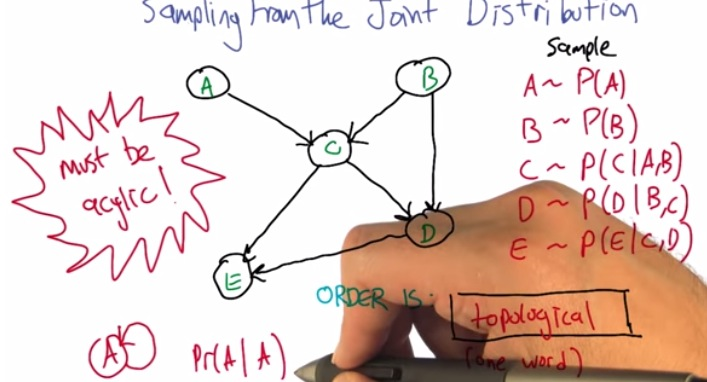• The word for this graph is topological.
• We need topological that in a sense, the order has to be acyclic. it has some  origin. Everything has come from something. Cyclic means it doesn't have origin.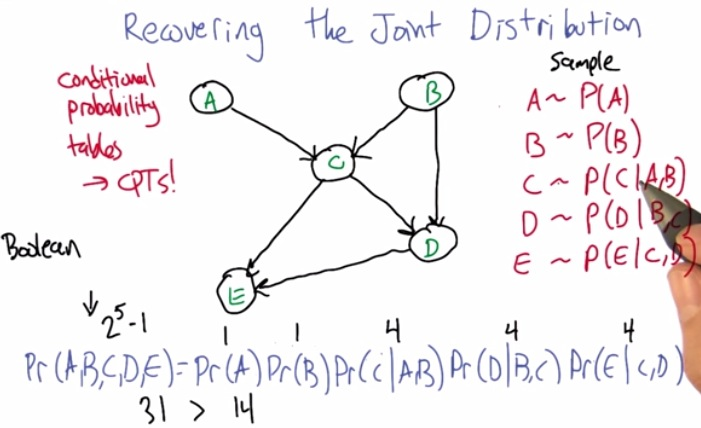• The more independent the node are, the simpler the algorithm. With this rule in red, we manage to have 4 or 1 combination.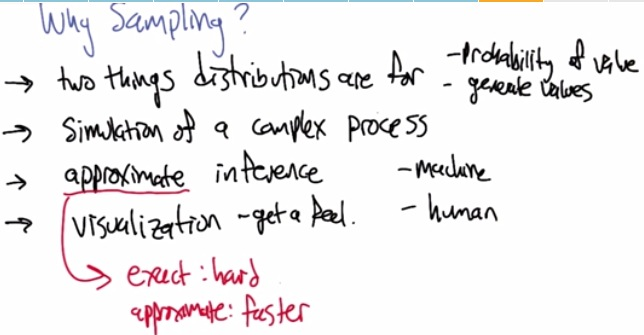• Why sampling matters?
• There's some huge value with complex relationship. We may want to sampling a bunch of them to make sense what usually can we infer from the data. This take into the notion of simulation of a complex process.
• Visualization: Because the data is lot and complex, sampling from the data can give us better instuition and insight what we can do to the data, which matters or not.
• Approximate inference: Why approximate? why not definite? Because it's much harder. We know that the data itself has many complex relationship, so it's pretty much impossible. And so making inference from date, approximately, can be a good job.
• By sampling mean, from the sampling, what are the weighted value of various kind of input, probability that one is higher than the other.